Download Presentation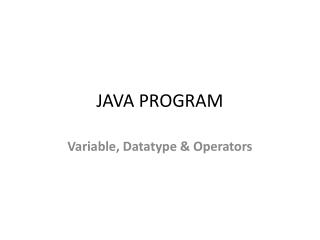JAVA PROGRAM

# JAVA PROGRAM - PowerPoint PPT Presentation

JAVA PROGRAM. Variable, Datatype & Operators. In this page, we will learn about the variable and java data types. Variable is a name of memory location. There are three types of variables: local, instance and static. There are two types of datatypes in java, primitive and non-primitive.I am the owner, or an agent authorized to act on behalf of the owner, of the copyrighted work described.
Download Presentation## JAVA PROGRAM

An Image/Link below is provided (as is) to download presentation

Download Policy: Content on the Website is provided to you AS IS for your information and personal use and may not be sold / licensed / shared on other websites without getting consent from its author.While downloading, if for some reason you are not able to download a presentation, the publisher may have deleted the file from their server.

- - - - - - - - - - - - - - - - - - - - - - - - - - E N D - - - - - - - - - - - - - - - - - - - - - - - - - -
Presentation Transcript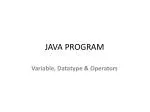### JAVA PROGRAM

Variable, Datatype& Operators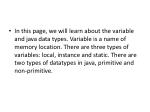In this page, we will learn about the variable and java data types. Variable is a name of memory location. There are three types of variables: local, instance and static. There are two types of datatypes in java, primitive and non-primitive.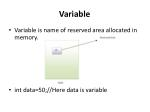Variable
• Variable is name of reserved area allocated in memory.
• int data=50;//Here data is variable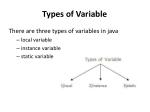Types of Variable

There are three types of variables in java

• local variable
• instance variable
• static variable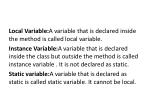Local Variable:A variable that is declared inside the method is called local variable.

Instance Variable:A variable that is declared inside the class but outside the method is called instance variable . It is not declared as static.

Static variable:A variable that is declared as static is called static variable. It cannot be local.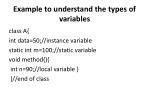Example to understand the types of variables

class A{

int data=50;//instance variable

static int m=100;//static variable

void method(){

int n=90;//local variable }

}//end of class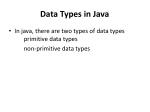Data Types in Java
• In java, there are two types of data types primitive data types

non-primitive data types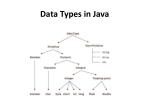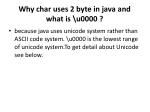Why char uses 2 byte in java and what is \u0000 ?
• because java uses unicode system rather than ASCII code system. \u0000 is the lowest range of unicodesystem.To get detail about Unicode see below.Operators in java
• Operator is a special symbol that is used to perform operations. There are many types of operators in java such as unary operator, arithmetic operator, relational operator, shift operator, bitwise operator, ternary operator and assignment operator.Useful Programs:
• There is given some useful programs such as factorial number, prime number, fibonacci series etc1) Program of factorial number.

class Operation{

static int fact(int number){

int f=1;

for(int i=1;i<=number;i++)

{

f=f*i;

}

return f;

}

public static void main(String args[]){ int result=fact(5); System.out.println("Factorial of 5="+result); } }2) Program of fibonacci series.

class Fabnoci{

public static void main(String...args) {

int n=10,i,f0=1,f1=1,f2=0;

for(i=1;i<=n;i++)

{ f2=f0+f1; f0=f1; f1=f2; f2=f0; System.out.println(f2); } } }3) Program of armstrong number.

class ArmStrong{ public static void main(String...args) { int n=153,c=0,a,d; d=n; while(n>0) { a=n%10; n=n/10; c=c+(a*a*a); } if(d==c) System.out.println("armstrong number"); else System.out.println("it is not an armstrong number"); } }4) Program of checking palindrome number
• class Palindrome { public static void main( String...args) { int a=242; int n=a,b=a,rev=0; while(n>0) { a=n%10; rev=rev*10+a; n=n/10; } if(rev==b) System.out.println("it is Palindrome"); else System.out.println("it is not palinedrome"); } }• class SwapTwoNumbers{ public static void main(String args[]){ int a=40,b=5; a=a*b; b=a/b; a=a/b; System.out.println("a= "+a); System.out.println("b= "+b); } }6) Program of factorial number by recursion
• class FactRecursion{ static int fact(int n){ if(n==1) return 1; return n*=fact(n-1); } public static void main(String args[]){ int f=fact(5); System.out.println(f); } }